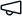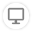Give us feedback# Expression Evaluator

Stable version 1.0.0 (Compatible with OutSystems 11)
Uploaded on 10 July 2019 by
5.0
(2 ratings)# Expression Evaluator

Details
Expression evaluator can parse any expression and evaluate the result, including static or dynamic parameters.

Expression evaluator can parse any expression and evaluate the result, including static or dynamic parameters.

Exp1.  - (2+3)*2 = 10

Exp2.  - (A*B*C)/D where you can set the value of variables like A,B,C,D and send it as parameter to the function to get the result.

Complex expression can be evaluated easily. Like - Round(Pow([X], 2) + Pow([Y], 2) + [Z], 2)

How to use:

Function 1: GetResultForExpression

A] Set the Expression parameter with the expression you want to evaluate, like (3+2)/4

B] Get the result from "Result" output parameter

Function 2: GetResultForExpressionWithParameter

A] You can set a dynamic expression like, (A+B+C)*D where you need to set the value of the

parametes for A,B,C,D as name value pair and add in the "NameValue" structure list. Now set

the input parameter "ParameterList" with this list.

B} Get the result from "Result" output parameter

Release notes (1.0.0)
Reviews (0)